Critical Points of a Time-Reversal Breaking Topological Insulator

Taylor L. Hughes May 30, 2008

(Submitted as coursework for Appied Physics 373, Stanford University, Spring 2008)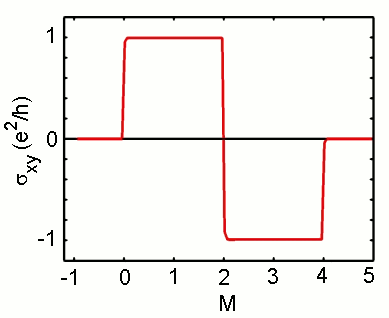Fig. 1: DC Hall conductivity of the topological insulator as a function of the Hamiltonian parameter M.

In this page we discuss a prototype model for a time-reversal symmetry breaking topological insulator. We will show that the model exhibits a quantum phase transition between a topologically trivial state and a non-trivial state characterized by a finite quantized Hall conductivity. After writing down the model we will discuss the generic insulating bulk bandstructure and the existence of chiral edge states in a strip geometry. Then we will show the existence of gap-closing phase transitions across which the Hall conductance jumps from zero to an integer value.

The prototype model considered here has two orbitals in (2+1)-d on a square lattice with nearest-neighbor hoppings. We can expand any two orbital model in the basis of Pauli matrices and the Hamiltonian is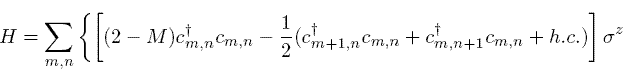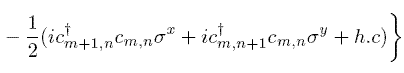where m,n are the lattice indices on the x,y directions respectively, and M is a parameter. With periodic boundary conditions we can diagonalize this model by Fourier transformation to get the bulk bandstructure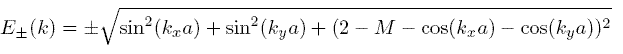where a is the lattice constant which will be set to 1 from now on. To gain intuition for this model one can expand it around kx=ky=0 and M=0 to find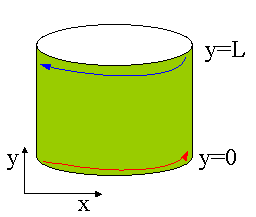Fig. 2: Strip geometry i.e. a cylinder. Blue and red circulating lines indicate chiral edge states.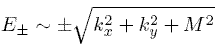which is the dispersion for a massive Dirac fermion in (2+1)-d. Thus at low energies our lattice model looks Dirac-like. At high energies the dispersion is no longer linear in k and the effects of the lattice regularization enter.

One can see that for values of M=0,2,4 the spectrum no longer has a gap. For example at M=0 we find E+(kx=0,ky=0)=E-(kx=0,ky=0) so the gap closes at (kx,ky)=(0,0). At M=2 the gap closes at two places (kx,ky)=(0,π),(π,0) and for M=4 the gap closes at (kx,ky)=(π ,π). In between these critical points the spectrum is insulating. One can pose an obvious question, is there any way to distinguish the insulating phases? The answer is yes and we will illustrate two common ways to do this which are intimately related.

Quantized Hall Conductivity

One can calculate the off-diagonal response of this model to an electric-field. The transport in this case is the Hall conductivity σxy. For this prototype model the calculation of the Hall conductivity is straightforward, but I will not reproduce it here. See the Refs. [1,2] for two different accounts. If we parameterize our Fourier transformed Hamiltonian via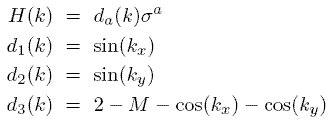then the DC Hall conductivity is given by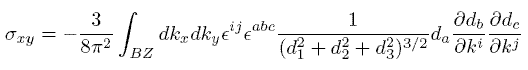where i,j=x,y and a,b,c=1,2,3, εijabc are the 2 and 3 index totally-antisymmetric tensors respectively, and the integral is over the first Brillouin zone. The value of σxy depends on the parameter M and we plot its value vs. M in Fig. 1.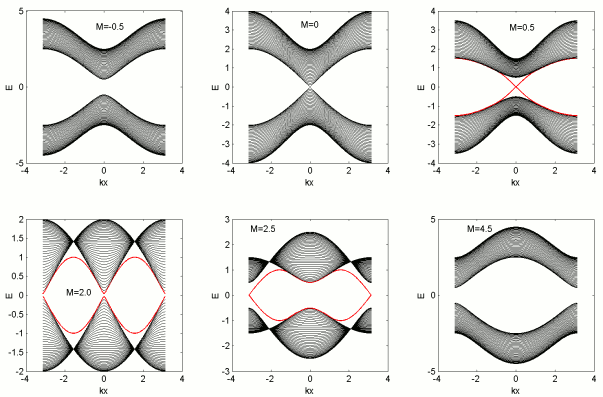Fig. 3: Band structure for strip geometry with L=40, for various values of the parameter M. The surface state is colored red. For M=-0.5 the system is an insulator with no Hall conductivity and no edge states. At M=0.0 the system is gapless and is at a critical point. At M=0.5 the system has one chiral edge state on each edge which indicates a Hall conductivity of 1 e2/h. At M=2.0 the system is gapless at two points and the Hall conductivity jumps by 2 to a value of -1 e2/h and again one chiral edge state on each edge. At M=4.0 (not shown) the system has another critical point and transitions into the last panel with M=4.5 which is again a trivial band insulator.

One can see that exactly at M=0,2,4, σxy jumps. At the first and last transition there is a jump of magnitude 1 while at the middle transition the jump is by 2. This difference is due to the fact that the amount of the jump is proportional to the number of gap closings. Thus the middle transition has two points where the gap closes and the Hall conductivity jumps by 2. This transport coefficient already gives us a physical way to distinguish the phases but we would like to illustrate a complementary view point.

Edge States

We want to study the Hamiltonian in a strip geometry shown in Fig. 2, with periodic boundary conditions along the x-direction, but open boundary conditions on the y-direction. This means that we terminate the lattice at y=0 and y=L so that it is finite in this direction. We can no longer diagonalize the Hamiltonian by Fourier transformation because the y-direction is no longer homogeneous. There is an easy way to solve this problem however. We simply Fourier transform only on the x-direction and numerically diagonalize the simple 1d model that is left over for each value of kx. The partially Fourier transformed Hamiltonian is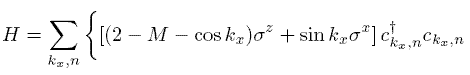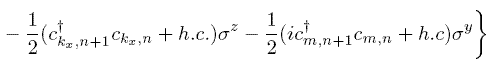We can solve the 1d tightbinding model for each value of kx and plot the spectrum vs. kx to get the bandstructure. We have done this in Fig. 3 for several values of M.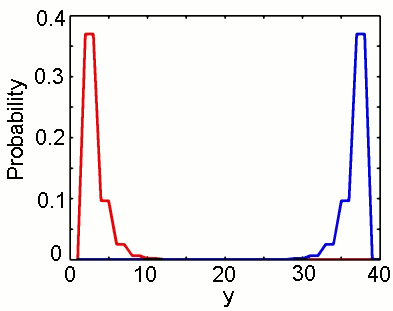Fig. 4: Square of the right-moving (red) and left-moving (blue) surface state wavefunctions for the case of M=5.

In Fig. 4 we plot the squared wavefunctions of left-moving and right-moving surface states for the case of M=0.5. Note that they lie on different edges. This gives the system a chirality and indicates that time-reversal symmetry has been broken. In fact, when the system is not gapless, time-reversal has been broken. Typically an energy crossing like the edge state crossing when M=0.5 is not generic, and the states will backscatter to open a gap. However, in this case, one state lies on one edge and the other on the opposite edge. This means that for any backscattering is supressed by the width of the sample. To open a gap the electron would have to hop from one edge to the other. Thus for edges which are far apart electrons travelling along the edge cannot be backscattered which leads to some interesting transport effects.

Source Code

The source code for all of the calculations done in this work can be found here. We used the GNU scientific library for the matrix diagonalization routine and complex numbers. This library is standard in all GNU Linux distributions and can be used on any platform including Windows via the Cygwin emulator.

© 2008 T. L. Hughes. The author grants permission to copy, distribute and display this work in unaltered form, with attribution to the author, for noncommercial purposes only. All other rights, including commercial rights, are reserved to the author.

References

 D. J. Thouless, M. Kohmoto, M.P. Nightingale, and M. den Nijs, Phys. Rev. Lett., 49,405(1982).

 X.-L. Qi, Y.-S. Wu, S.-C. Zhang, Phys. Rev. B 74,085308(2006).